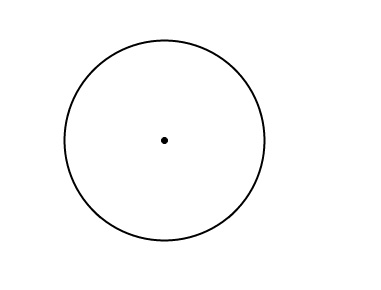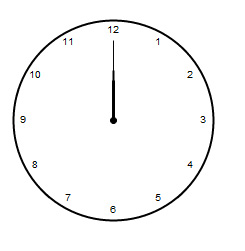# Monday Fun: Creating a Clock Animation Without CSS3

Monday seems to be the most boring day, starting a new week can be really tough. So we will start with something fun and exciting, today we are going to create a clock animation that works in all browser, no CSS3. So Let’s get started.

### Step 1 – Setting up workspace

For our little animation effect we are going to use Raphael library, you can get it from here. Now First create a html page and import the Raphael javascript file.

### Step 2

After that you have created the html page and imported Raphael.js. Create a new javascript and name it clock.js. Here we will code the animation effect. Now just add a div which will act as stage for our clock and that’s it for the html page. So far our code looks like –

```<html>
<script type="text/javascript" src="js/raphael-min.js"></script>
<script type="text/javascript" src="js/clock.js"></script>

<title>Clock</title>
<body>
<div id="pane" ></div>

</body>
</html>
```

### Step 3

First we are going to create a canvas using Raphael, wrap it in the window onload function. Canvas is created by calling Raphael() , which creates a Raphael object which we will use it for future reference when we will call other methods.

```window.onload = function(){

var canvas = Raphael("pane",0,0,500,500);
};
```

Here using Raphael’s function we have converted the div with id pane into an canvas with coordinates 0,0 and with dimensions 500 by 500.

### Step 4

Creating a skeleton, now we are going to create a basic structure of our clock, for that we will two concentric circles with outer circle’s radius will be our clock’s size and inner clock’s circle will be a reference point.

```canvas.circle(200,150,100).attr("stroke-width",2);
canvas.circle(200,150,3).attr("fill","#000");
```### Step 5

Now we are going to add numbers along the side of our clock’s circumference. For that, we will be using little maths. First we will declare variables –

• cx,cy are center x and y of the imaginary circle on which circumference’s text will be situated.
• startangle is the position of the first text, angle is the gap between two text nodes. Here we have 12 hours divide by 360 that is 30.
• endangle will serve as reference for next text position.
```var rad = Math.PI / 180,
cx = 200,cy =150 ,r = 90,
startangle = -90,angle=30,x,y, endangle;

for(i=1;i<13;i++)
{

endangle = startangle + angle ;

x = cx + r  * Math.cos(endangle * rad);
y = cy + r * Math.sin(endangle * rad);

canvas.text(x,y,i+"");

startangle = endangle;
}
```

Then we will loop from 1 to 12 calculate x,y position and finally insert the number at it’s position.### Step 6 – Adding hands

So for we have added the numbers, now time to add the second, minute and hour hand. This can be done by creating paths from starting point from the center of the circle, to the length depending on each hand.

```var hand = canvas.path("M200 70L200 150").attr("stroke-width",1);
var minute_hand = canvas.path("M200 100L200 150").attr("stroke-width",2);
var hour_hand = canvas.path("M200 110L200 150").attr("stroke-width",3);
```### Step 7 – Setting up Current time

Our clock has now all it’s parts. Time to set it to the the current time. For this, we will retrieve the current date using date object and retrieve current seconds, minutes and hours.

```var time = new Date();
angle = time.getSeconds() * 6;
minute_hand.rotate(6 * time.getMinutes(),200,150);

var hr = time.getHours();
if(hr>12)
hr = hr -11;
hour_hand.rotate(30 * hr,200,150);
```

After that we are going to rotate each have hand based on time. For minute hand one minute takes 6 degrees, so we will multiply current minutes by 6. For hour in our clock it
takes 30 degrees so will multiply current hour by 30. There is different in degrees because there are 5 minutes between two numbers and only 1 hour for the consecutive one.### Step 8 – Finally making the clock tick

Finally making the clock tick, to animate the clock we will using setInterval function and rotate each hand by defined amount of degrees

• For second hand we will move it by 6 degrees. If angle count goes ove 360 we will reset it.
• For minute hand whenever second is over( angle > 360 ) we will rotate minute hand by 6 degrees.
• Finally we will slowly move the hour hand by a very small degree.
```var minute_angle= 6 + time.getMinutes()*6,hour_angle=0.5+ time.getMinutes()*30;
setInterval(function(){
angle = angle + 6;
if(angle>=360)
{
angle=0;

minute_hand.rotate(minute_angle,200,150);
minute_angle = minute_angle + 6;

hour_hand.rotate(hour_angle,200,150);
hour_angle = hour_angle + 0.5;
}
if(minute_angle>=360)
{
minute_angle=0;

}

hand.rotate(angle,200,150);

},1000);
```

### Step 9 – Putting it altogether

Finally our code looks like.

```window.onload = function(){

var canvas = Raphael("pane",0,0,500,500);

canvas.circle(200,150,100).attr("stroke-width",2);
canvas.circle(200,150,3).attr("fill","#000");

var angleplus = 360,rad = Math.PI / 180,
cx = 200,cy =150 ,r = 90,
startangle = -90,angle=30,x,y, endangle;

for(i=1;i<13;i++)
{

endangle = startangle + angle ;

x = cx + r  * Math.cos(endangle * rad);
y = cy + r * Math.sin(endangle * rad);

canvas.text(x,y,i+"");

startangle = endangle;
}

var hand = canvas.path("M200 70L200 150").attr("stroke-width",1);
var minute_hand = canvas.path("M200 100L200 150").attr("stroke-width",2);
var hour_hand = canvas.path("M200 110L200 150").attr("stroke-width",3);

var time = new Date();

angle = time.getSeconds() * 6;

minute_hand.rotate(6 * time.getMinutes(),200,150);

var hr = time.getHours();
if(hr>12)
hr = hr -11;

hour_hand.rotate(30 * hr,200,150);

var minute_angle= 6 + time.getMinutes()*6,hour_angle=0.5+ time.getMinutes()*30;
setInterval(function(){
angle = angle + 6;
if(angle>=360)
{
angle=0;

minute_hand.rotate(minute_angle,200,150);
minute_angle = minute_angle + 6;

hour_hand.rotate(hour_angle,200,150);
hour_angle = hour_angle + 0.5;
}
if(minute_angle>=360)
{
minute_angle=0;

}

hand.rotate(angle,200,150);

},1000);

};
```

Hope you enjoyed the tutorial, works on all browsers. Be sure to check out the demo, you can use it instead of a flash with a little more styling.

Sign up for Testking 70-433 online web designing course and download Testking 70-294 CSS tutorials and Testking 642-373 videos to learn how to create inspiring designs for your web project.

This entry was posted on Monday, May 24th, 2010 at 14:54 and is filed under Tutorials. You can follow any responses to this entry through the RSS 2.0 feed. You can leave a response, or trackback from your own site.

Hi, I am Abhin from beautiful city of lakes Udaipur, India. When it comes to work, I have an obsessive compulsive disorder to do everything I find and most of the time, I end up doing nothing ;). UI/UE fascinates me.

### 4 Responses »

1. haha nice!

when I get some time I’m going to give this a shot!

Cheers Abhin!

2. It’s nice and good usage of Raphael lib, but it would be even better if the hour pointer can rotate bit by bit according to how many the minute has elapsed.

3. It�s nice and good usage of Raphael lib, when I get some time I�m going to give this a shot!
thanks for sharing

4. Hello

I need to rotate a text runtime on button click event.please provide me any help regard this.i m waiting for ur reply.
Thanks
rotation angle 0,20,40,90 etc